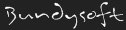L3DT documentationLarge 3D terrain generator

# Differences

This shows you the differences between two versions of the page.

zeolite:types:vector4f [2017/08/31 04:49] (current)
Line 1: Line 1:
+====== vector4f ======

+===== Description =====
+
+''vector4f'' is structure used to describe a 4-dimensional vector in 32-bit floating point precision.
+
+Vectors may or may not be normalised.
+
+===== Definition =====
+
+//In Zeolite_defines.h: //
+
+<code="C">
+typedef struct {
+  float a;
+  float b;
+  float c;
+  float d;
+} vector4f;
+</code>
+
+===== Member data =====
+
+^ Member name ^ Type ^ Comments ^
+|  //a//  | float | The first vector component. |
+|  //b//  | float | The second vector component. |
+|  //c//  | float | The third vector component. |
+|  //d//  | float | The fourth vector component. |
+
+
+===== Type identifiers =====
+
+^ [[zeolite:VarID]] | VarID_vector4f |
+^ [[zeolite:MapID]] | N/A (vector4f is not a valid map type.) |